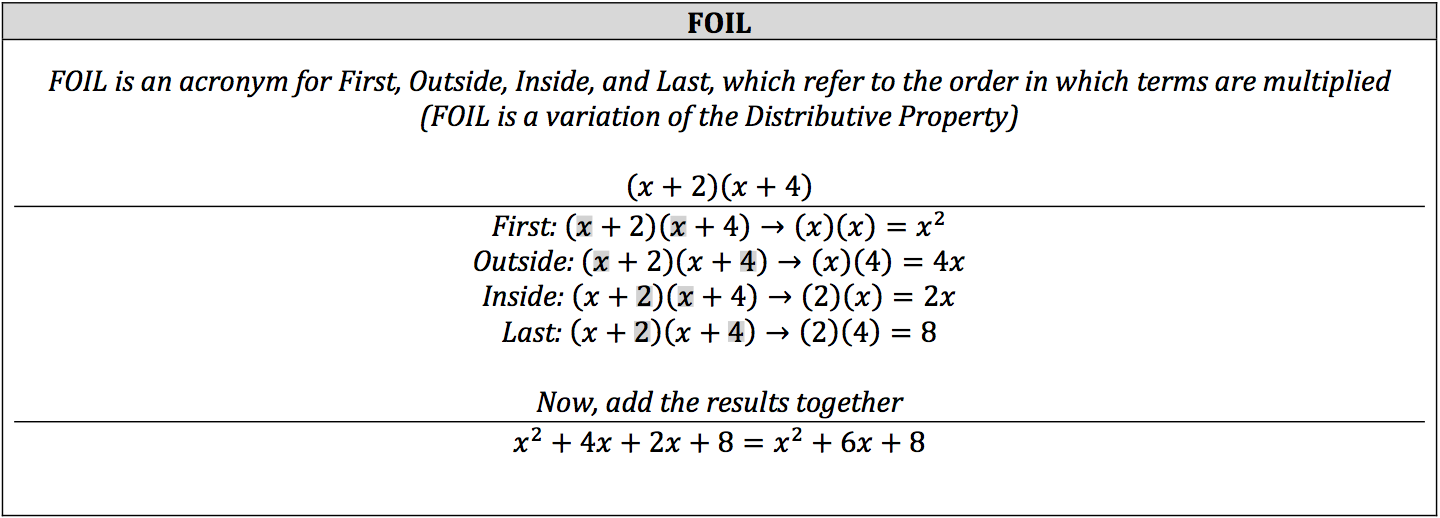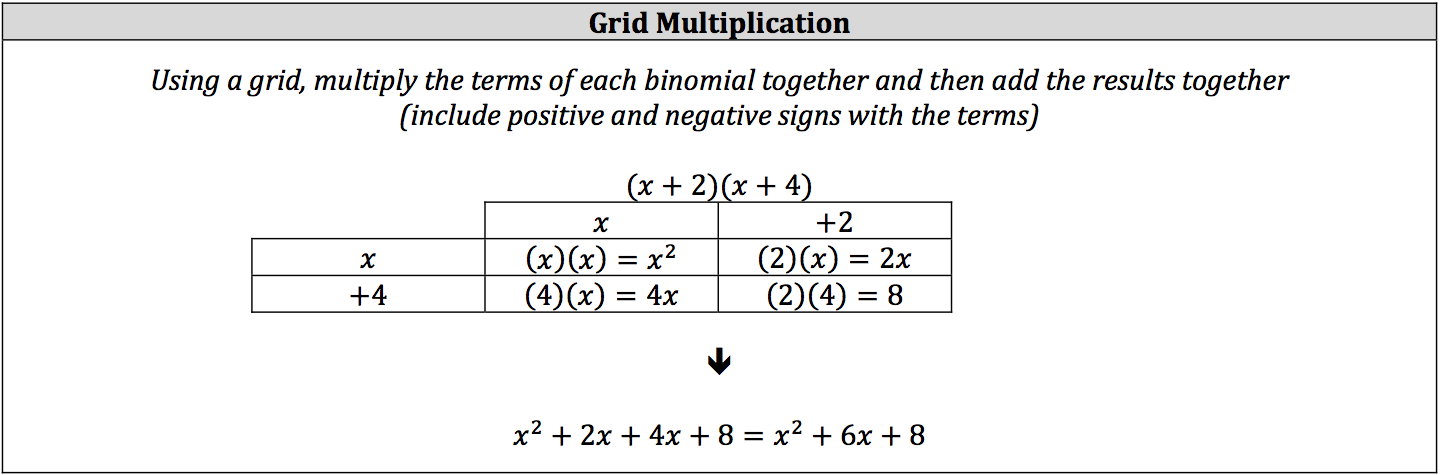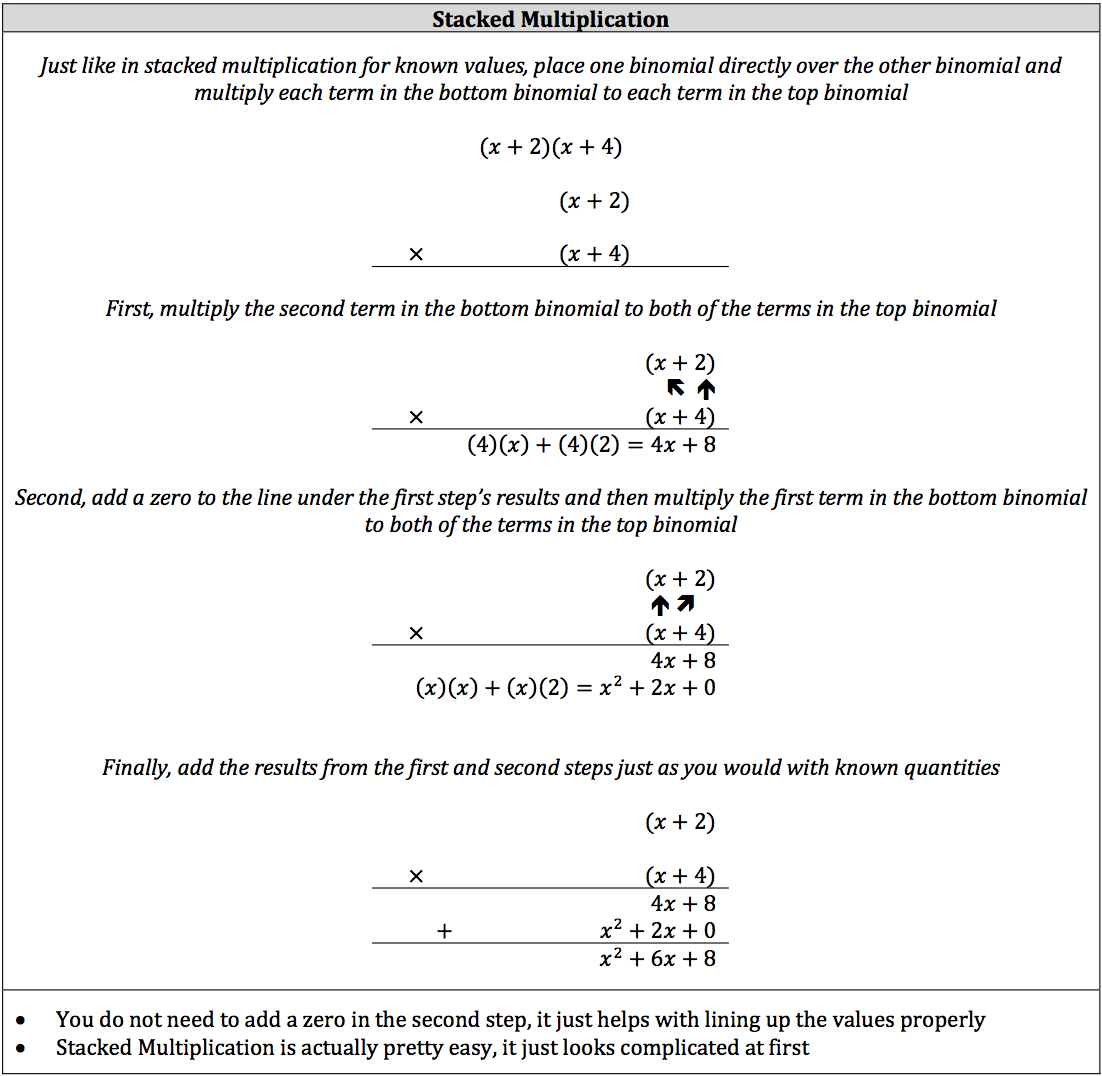Algebraic expressions are not solely made up of coefficients and a single variable. Algebraic expressions can be made up of multiple terms, which are either added or subtracted from each other. These terms can include several distinct variables, their coefficients, and known quantities called constants. Depending on their number of terms, algebraic expressions can be labeled as a monomial, binomial, trinomial, or a polynomial.

### Definition of Monomial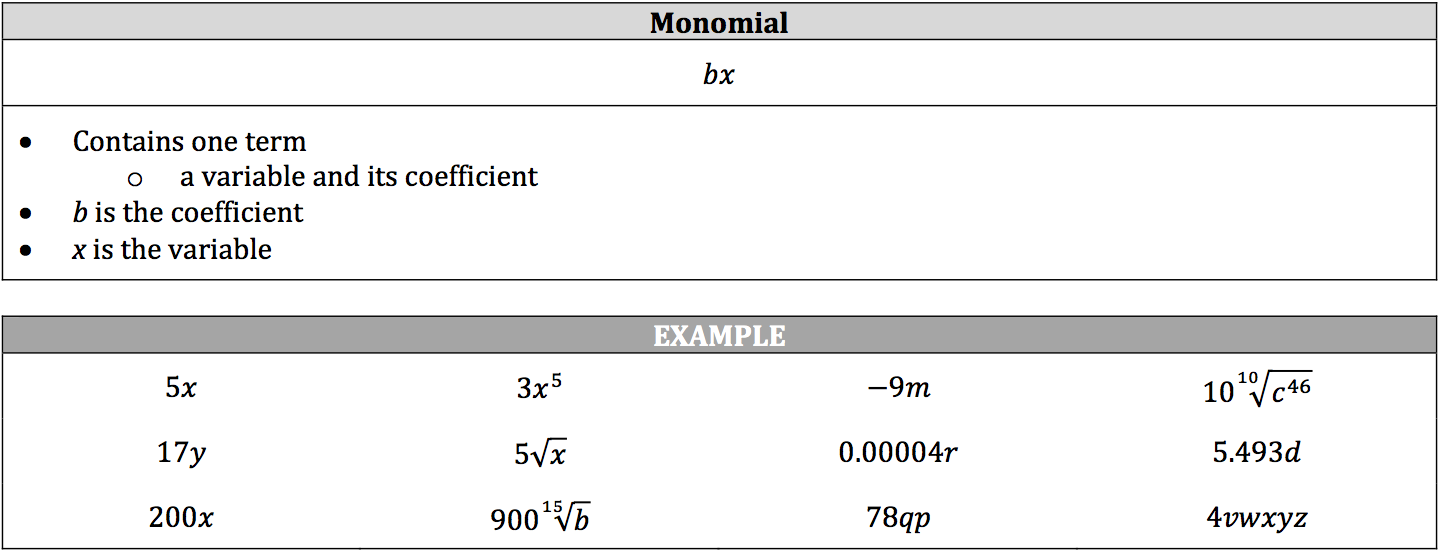### Definition of Binomial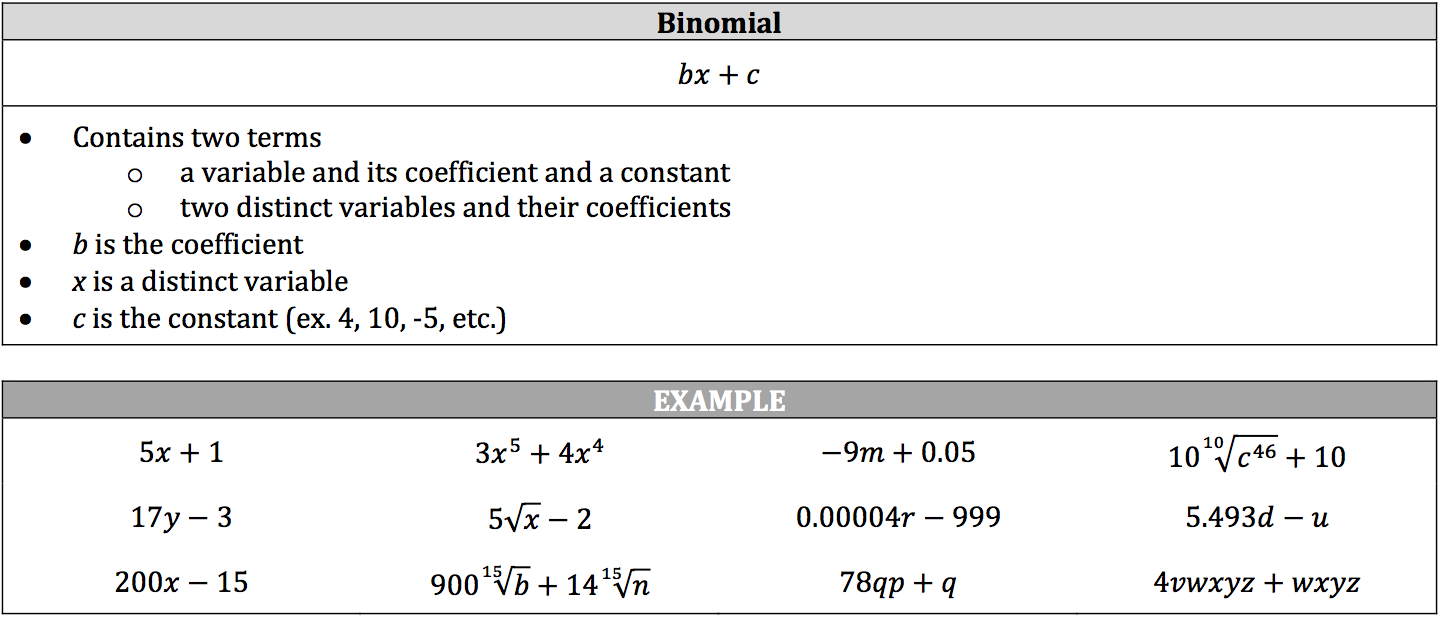### Definition of Trinomial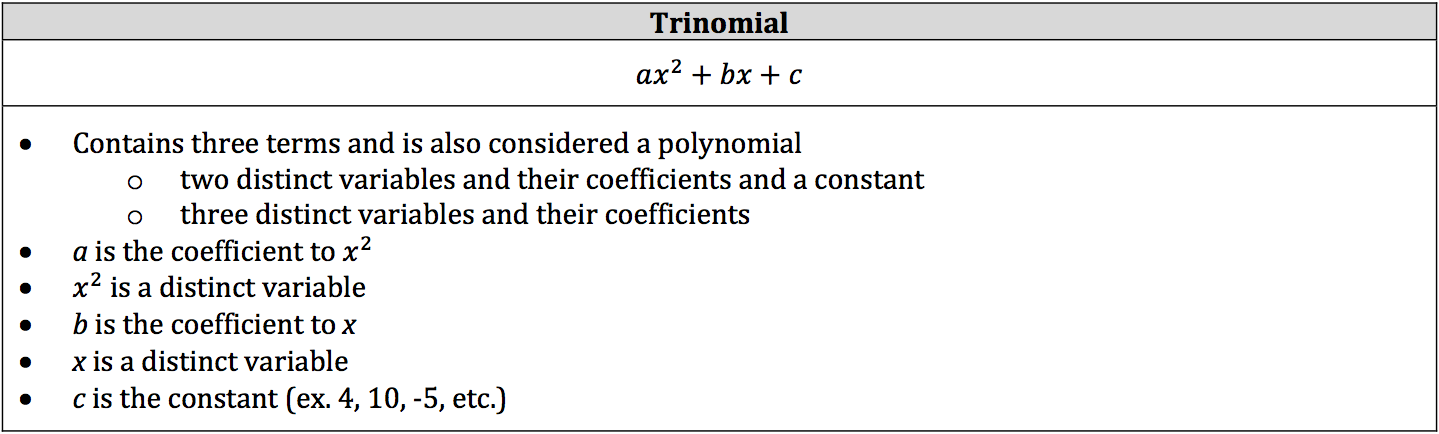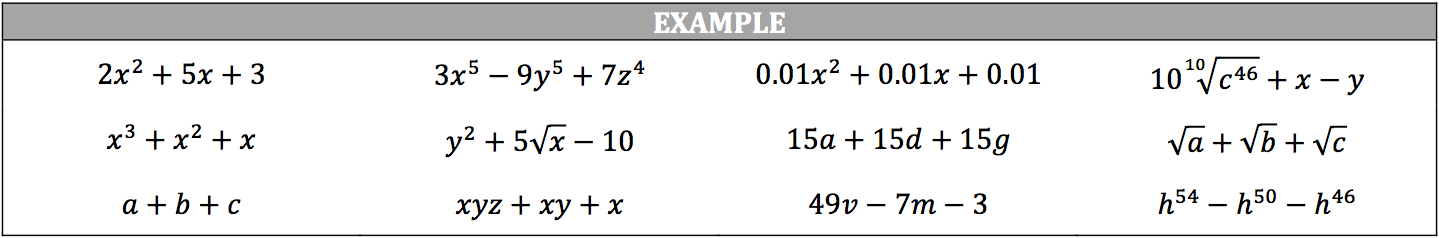### Definition of a Polynomial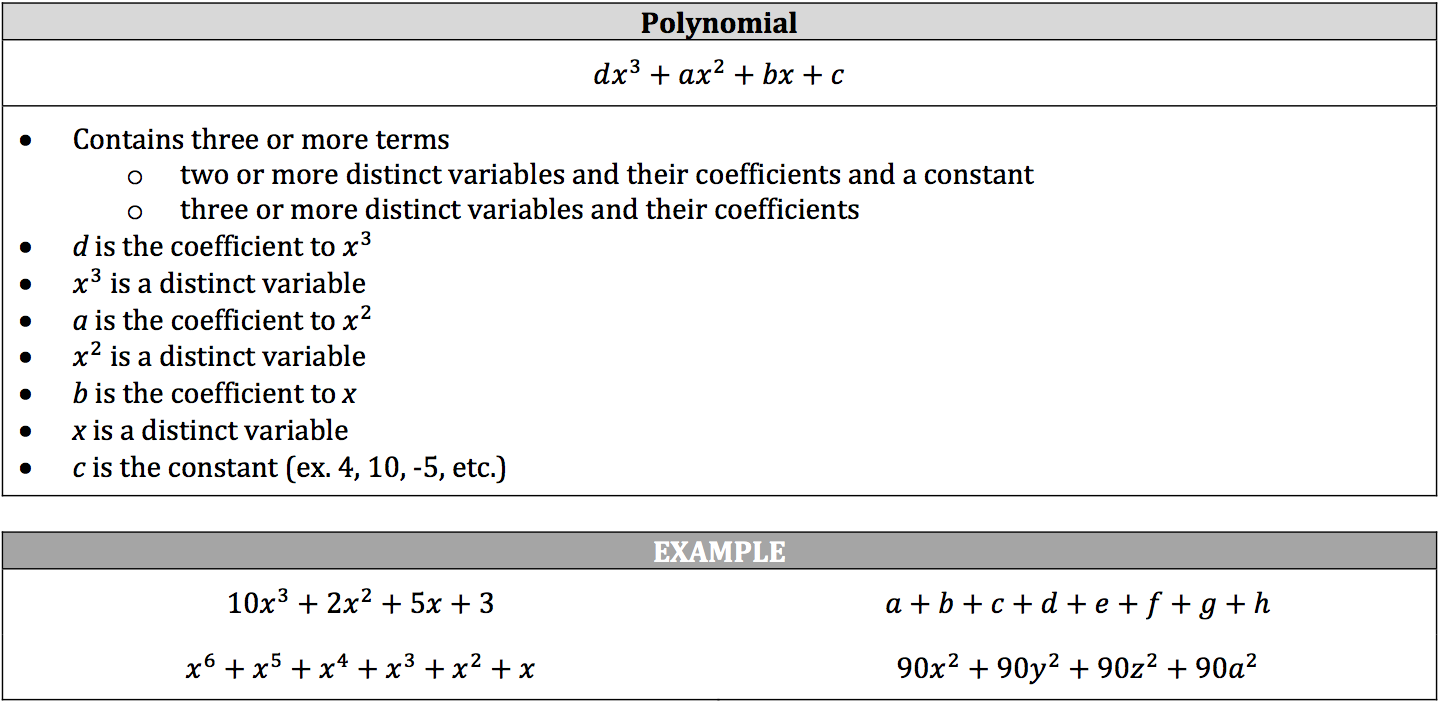### Multiplying Polynomials

You can multiply polynomials using the :

• distributive property
• FOIL
• grid multiplication
• stacked multiplication

These four methods all do the same thing; they’re just presented in different ways. Use the method that is easiest for you to remember. We’ll focus more on multiplying binomials with the same variable, but the methodologies are the same for polynomials.